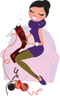#Crochet Pattern Central# Crochet Abbreviations

 alt = alternate approx = approximately BP = back post BLO = back loop only BPdc = back post double crochet beg = begin, beginning CC = contrasting color ch = chain cl = cluster cont = continue dbl = double dtr or dbl tr = double triple dc = double crochet dec = decrease dir = directions dk = dark dnt = do not turn ea = each ex sc = extended single crochet fig = figure 1 (picture of stitch) FLO = front loop only FP = front post FPdc = front post double crochet fig = figure 1 (picture of stitch) fo = finish off foll = following gm or gr = grams gr = group hdc = half double crochet hk = hook htr = half triple crochet in = inch inc = increase incl = including inst = instructions lp(s) = loop(s) lt = light MC = main color med = medium mm = millimeter no. = number oz(s) = ounce pat st = pattern stitch pc st = popcorn stitch prev = previous rem = remaining rep = repeat rnd = round rs = right side sc = single crochet sk = skip sl st = slip stitch sp = space st(s) = stitch(es) tch = turning chain tog = together tr = triple (treble) x times = (such as repeat 5x) ws = wrong side yo = yarn over

Home | CPC's Free Patterns | Site Map | About CPC | Link to CPC | FAQs | Privacy Policy | Contact Me